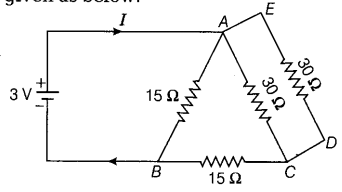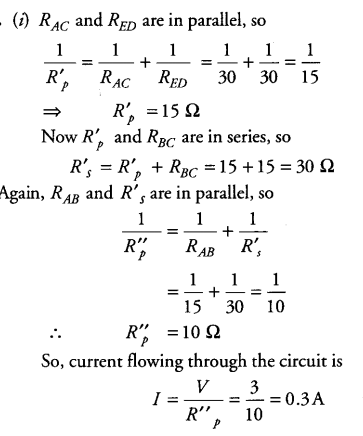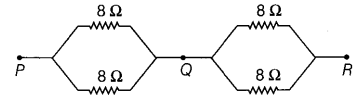# (i) Find the value of current I in the circuit given as below:

(i) Find the value of current I in the circuit given as below:(ii) You have four resistors of 8 \Omega each. Show how would you connect these resistors to have effective resistance of 8 \Omega ?

(i)(ii) Two 8 \Omega resistors are connected in parallel. Two such parallel combination must be connected in series to get effective resistance of 8 \Omega .
Such combination is shown as below: